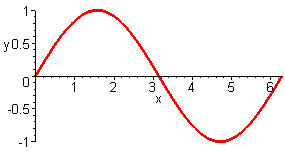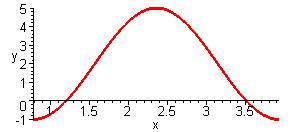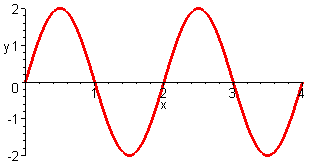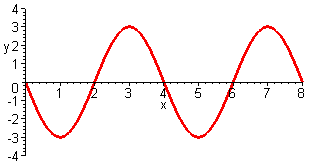# Math 1050 - MAPLE LAB IV

Maple Lab IV

## Open configuration options Math 1050 - Precalculus - Trigonometric Functions

Graphing Trigonometric Functions

Sine- The standard sine function has an amplitude of 1 and a period of 2p. The graph of f(x)=asin(bx+c)+d, is the sine wave translated and stretched/shrank. This graph now has an amplitude of |a|, a period of 2p / |b|, and the d represents a vertical shift in the graph. If a is negative, then the graph is reflected about the x axis. The graph also experiences horizontal shifts and stretches as a result of the b and c. One full period usually ranges from 0 to 2p, now the angle is bx+c so let's find where it is now located. If 0 < bx+c < 2p  then -c < bx < -c+2p  and -c/b < x < -c/b+2p/b. So the period of the graph that use to begin at the origin and end at 2p, now begins at -c/b and ends at -c/b+2p/b, this is the phase shift. Let's plot one period of the graphs sin(x) and 2sin(4x+p)-1. First find the amplitude, period, and phase shifts for each graph. The amplitude of sin(x) is 1 so we need to display from -1 to 1 on the y axis, the period of the standard sin(x) function is 2p, and there is no phase shift so we can graph from 0 to 2p on the x axis. For the second function the amplitude is 2 (from a=2), then the graph is moved down one (from d= -1) so we need to show at least from -3 to 1 on the y axis, the period is of length p/2 (from 2p/|b|, b=4), the phase shift tells us the graph begins at - p /4 and ends at p /4 (from -c/b and -c/b+2p/b, b=4, c= p ) so we need to graph from - p /4 to p /4 on the x axis.

> plot(sin(x),x=0..2*Pi,y=-1..1);> plot(2*sin(4*x+Pi)-1,x=-Pi/4..Pi/4, y=-3..1);Notice that MAPLE measures all the values as rational numbers, so p /4 is approximately 0.8 on the x axis. Also note that in MAPLE you must type in p as the word Pi with a capital P, if you use a lower case p it will print the letter form of pi ( p ) and not understand it as a numerical value.

Cosine- The standard cosine function also has an amplitude of 1 and a period of 2p. All other shifts and transformations of acos(bx+c)+d are found exactly the same as for the sine function. Graph cos(x) and -3cos(2x- p /2)+2. The first graph will have the standard amplitude of 1, so we need to display from -1 to 1 on the y axis, and a period of 2p  with the graph beginning at 0 ending at 2p on the x axis. The graph of -3cos(2x- p /2)+2 has an amplitude of |-3| =3 (a= -3) then it is shifted up vertically by 2 (d=2) so we need to display the y axis from -1 to 5. The period is of length p (2p / |b| , b=2) and the graph now begins at p /4 (-c/b, c= -p/2) and ends at 5p /4 (-c/b+2p /b) so we need to display the x axis from p /4 to 5p /4. This graph should also appear reflected about the x axis since a is negative.

> plot(cos(x),x=0..2*Pi, y=-1..1);> plot(-3*cos(2*x-Pi/2)+2,x=Pi/4..5*Pi/4,y=-1..5);Tangent- The standard tangent function has a period length of p , with vertical asymptotes located at - p /2 and p /2, since this is where cos(x)=0. However the shifts and transformations of the function atan(bx+c)+d differ from those of the sine and cosine functions. The period of the tangent function is p /|b|, the point normally located at the origin is translated to -c/b on the x axis, and the asymptotes are moved to -c/b- p /(2b) and -c/b+ p /(2b). The a stretches the graph vertically, and d translates the graph vertically. Graph tan(x) and 2tan(3x- p )-4. The graph of tan(x) has a period of p that begins at -p /2 and ends at p /2, so we can display at least this on the x axis, and the range of the tan(x) is all real numbers so I will just display from -10 to 10 on the y axis. The graph of 2tan(3x- p )-4 will also have an infinite range so we can use the same y values, but the period is now of length p /3 (b=3), it begins at p /6 and ends at p /2 (b=3, c= -p ) if we display p /6 to p /2 on the x axis it will show one period.

> plot(tan(x),x=-Pi/2..Pi/2,y=-10..10);> plot(2*tan(3*x-Pi)-4,x=Pi/6..Pi/2,y=-10..10);Cotangent - The standard cotangent function is the reciprocal of the tangent and has a period length of p , with vertical asymptotes located at 0 and p , where sin(x)=0. However the shifts and transformations of the function acot(bx+c)+d also differ from those of the sine and cosine functions. The period of this cotangent function is p /|b|, the point normally located at the origin is translated to -c/b on the x axis, and the asymptotes are moved to -c/b and -c/b+p /b. The a stretches the graph vertically, and d translates the graph vertically. Graph cot(x).

> plot(cot(x),x=0..Pi,y=-10..10);Cosecant- The cosecant function is the reciprocal of the sine and has a period of 2p. However it has asymptotes where sin(x)=0, at 0, p and np , and an infinite range. The graph of acsc(bx+c)+d will have a vertical stretch of a and a vertical translation of d. The period for the cosecant like the sine is 2p /|b|, and the asymptotes will be located at -c/b and -c/b+ p /b, and one period of the graph will begin at -c/b and end at -c/b+2p /b. Graph csc(x).

> plot(csc(x),x=0..2*Pi, y=-3..3);Secant- The secant function is the reciprocal of the cosine and has a period of 2p. However it has asymptotes where cos(x)=0, at p/2, 3p/2 and p /2+np , and an infinite range. The graph of asec(bx+c)+d will have a vertical stretch of a and a vertical translation of d. The period for the secant like the cosine is 2p/|b|, and the asymptotes will be located at -c/b+ p /(2b) and -c/b+3p /(2b), and one period of the graph will begin at -c/b and end at -c/b+2p /b. Graph sec(x).

> plot(sec(x),x=0..2*Pi,y=-3..3);Exercises:

1. For the following functions find and state the amplitude and period length, and location of asymptotes if applicable. Then graph one full period using the phase shift to find the beginning and ending points of the period to display on the x axis, and the amplitude and vertical shift to determine the values needed for the y axis.
A. 3sin(2x- p )+1
B. 2cos(3x+ p /2)+2
C. -cos( p x- p /6)+1
D. tan(3x- p /6)

2. For each of the following graphs find an equation to represent it, then check your equation by graphing it (print this graph to turn in as well).
A.B.